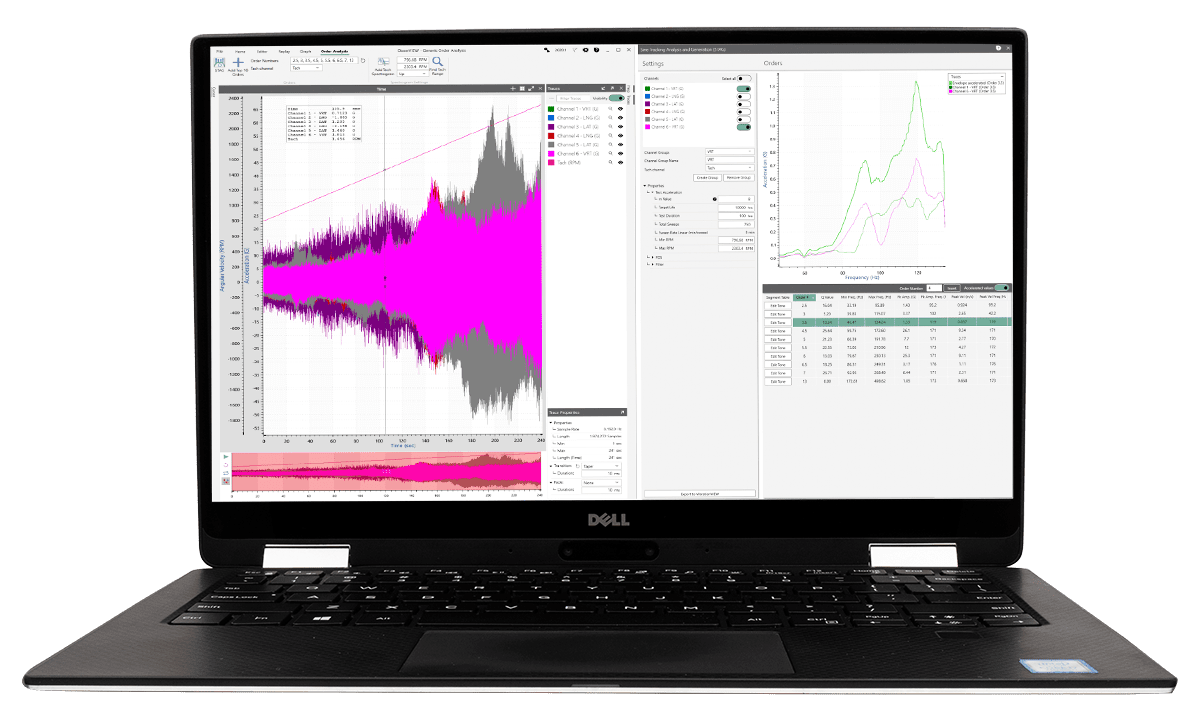# Vibration Test Acceleration

#### Test Generation, Vibration Control

Products are likely to fail when subject to consistent vibration. When the failure will occur, however, depends on several factors. To ensure the safety and longevity of a product, engineers must determine how long the product can withstand a specified vibration environment. Still, a well-built product may experience hundreds to thousands of hours of use. Therefore, engineers require a vibration testing method to assess a product over many hours without expending significant time to do so.

Using vibration test acceleration, a product can be tested for years of service in a fraction of the time. Depending on the vibration environment, the test acceleration can be performed in one of several ways. Today, the Fatigue Damage Spectrum (FDS) is a common method to accelerate a random vibration test. Still, as discussed later, there is a particular vibration environment where FDS is not entirely sufficient.

### Vibration Test Acceleration with the Fatigue Damage Spectrum (FDS)

The FDS is based on the Miner’s rule of cumulative damage, which states that a product will experience failure when it reaches its maximum amount of fatigue damage. A product is likely to experience a range of vibration environments in its lifetime and, in many cases, a vibration environment will be more complex than a simple sinusoid or Gaussian random waveform.

The FDS can be used to incorporate all vibration environments to create a true-to-life test. The FDS is calculated using a time domain analysis that includes rainflow analysis and cycle counting. Using the time domain analysis and a defined material property (m), the relative damage can be calculated and an FDS can be created. For test purposes, the FDS can then be converted into a PSD using Henderson-Piersol’s fatigue calculation method .

Rather than the Fast Fourier Transform (FFT), the FDS is based on the response of single degree-of-freedom (SDOF) systems. Altogether, the FDS is a reliable method for generating a random test reflective of the real world, which is often non-Gaussian and non-stationary.

### Parameters to Consider When Accelerating a Test Using the FDS

#### Quality Factor (Q)

The quality factor determines the damping value of an oscillation. A higher Q value will result in a lower rate of energy loss and, therefore, a slower end to the oscillation. Conversely, a lower Q value will result in a higher rate of energy loss and a faster end to the oscillation. The resonance quality factor is 0.5 divided by the critical damping ratio (ζ).

In FDS analysis, the quality factor is related to the Q of the filters used to generate the single/narrow band time waveforms. This value should be set greater than or equal to the sharpest resonance of the product within the test frequency range.

#### Slope of the S-N Curve (m)

The S-N curve displays an inverse relationship between the maximum applied stress (S) and the number of cycles to failure (N) on a logarithmic scale. A cyclical stress above the yield stress can result in low cycle fatigue (LCF) and a cyclical stress below the yield stress can result in high cycle fatigue (HCF).

The value of m is obtained from the S-N curve. MIL-STD-810H (2019) recommends using an m value of 7.5 for random vibration excitations, “but values between 5 and 8 are commonly used.” However, a value can be derived by testing several units with several different vibration levels until failure, and then finding the value of m that is most suitable for the data.

#### Kurtosis

Shakers running periodic random excitations can display non-Gaussian vibration responses in structural resonances. Kurtosis is used to describe a non-Gaussian random process. If the kurtosis of a random signal exceeds the Gaussian value of 3, the damage-inducing potential of the profile increases.

The FDS import assumes a Gaussian distribution of data because the Piersol method of computing fatigue converts the FDS back into a power spectral density (PSD) using a Gaussian distribution. The PSD is based on the relative damage calculation computed for each frequency and, by default, will be a Gaussian distribution. Therefore, the generated test will not have the same peak distribution as the real-world environment. Kurtosis control, such as Kurtosion®, can be added to modify the distribution of peaks so it is similar to the real-world environment while still maintaining the damage based on the computed FDS, life duration, and desired test duration.

When kurtosis is added to the FDS software, the overall GRMS level of the test is decreased. The duration of the test is a set value, and the lifetime fatigue value is not impacted. However, the distribution of peaks is different, so the overall GRMS of the test is lowered. In the end, the damage is still equivalent to the imported waveforms.

### Vibration Test Acceleration and Dominant Sinusoidal ComponentsWhile the FDS is the standard tool for vibration test acceleration, there is an environment where the FDS falls short. If a product is subject to an environment with dominant sinusoidal components, a Sine-on-Random (SoR) test—not a random test—should be employed. In such instances, the sine tones should be accelerated separately from the random energy that is accelerated with the FDS. Only after can the accelerated sine and random components be combined.

Vibration Research developed the Sine Tracking, Analysis and Generation (STAG) tool to create a Sine-on-Random test reflective of environments with rotational components, such as in engines. If the FDS is used for such an environment, then the dominant sine tones may not be represented accurately.

The STAG tool is an accurate and convenient method for developing an SoR test. It was developed to reduce the standard processing time and bypass manual entry for the highest-level of accuracy.

### Conclusion

Vibration test acceleration saves time and costs compared to testing at normal conditions. Being attentive to the characteristics of the vibration environment(s) will lend to a more accurate accelerated test.

For a more detailed description of accelerated vibration testing, download the paper How Do I Measure the Life of My Product?

#### References

 Henderson, G. R. & Piersol, A. G. (1995). Fatigue Damage Related Descriptor for Random Vibration Test Environments. Sound and Vibration, 21.

 Jiang, Y., Yun, G. J., Zhao, L., & Tao, J. (2015). Experimental Design and Validation of an Accelerated Random Vibration Fatigue Testing Methodology. Shock and Vibration, (147871), 1-13. doi:10.1155/2015/147871

Date

June 18, 2020

Author

Cherie Stoll

Category

Test Generation, Vibration Control